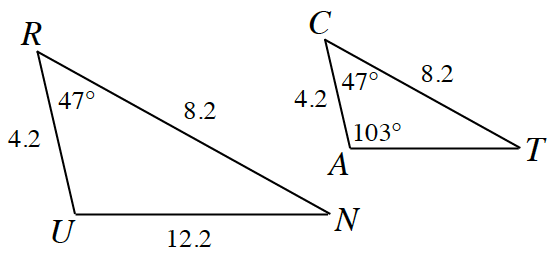### Home > GB8I > Chapter 7 Unit 8 > Lesson INT1: 7.1.7 > Problem7-79

7-79.

Are the two triangles at right congruent? Make a sketch of both triangles. If they are congruent, describe a sequence of rigid transformations that maps one onto the other. Note: The triangles are not drawn to scale.

Using the three rigid transformations: translation, rotation, and reflection, how can you get $ΔRUN$ to coincide (overlap) $ΔCAT$?

Read the Math Notes box in Lesson 7.1.7, review the conditions for Triangle Congruence.
Do these triangles have enough congruent parts in the correct order to be considered congruent?